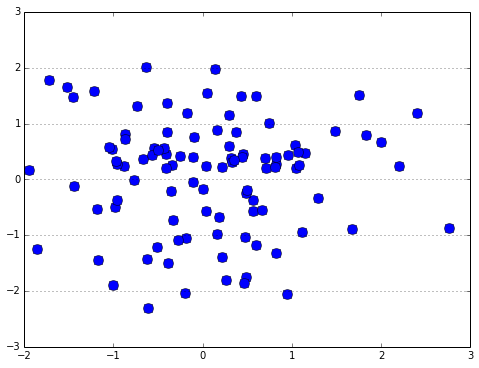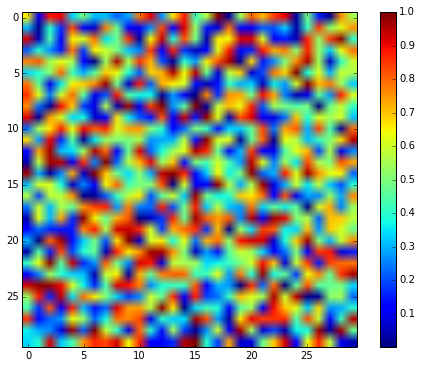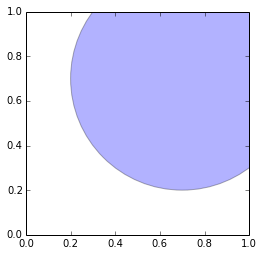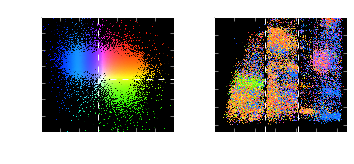# Visualization in Python: Matplotlib and Beyond¶

In :
%run talktools
%matplotlib inline


## Matplotlib sometimes gets a bad rap...¶

### First because of its defaults...¶

In :
import numpy as np
import matplotlib.pyplot as plt

x, y = np.random.normal(size=(2, 100))

fig = plt.figure(figsize=(8, 6))
plt.plot(x, y, 'ob', ms=10)
plt.grid(axis='y')## Wait... this reminds me of something...¶See this Excel vs. Python vs. IDL on the blog If We Assume for more side-by-side comparisons.

### It has horrible default color schemes...¶

In :
plt.figure(figsize=(8, 6))
plt.imshow(np.random.random((30, 30)))
plt.colorbar();### Matplotlib also draws flak for its static output in the notebook...¶

In :
ax = plt.axes(aspect='equal')(Click and drag? Who needs to click and drag?)

## But with enough effort...¶

you can make some halfway decent graphics...Asteroids from the Sloan Digital Sky Survey

But this takes a lot of by-hand tweaking...

In :
# let's load the source which generates this graphic:


In :
"""
SDSS Stripe 82 Moving Object Catalog
------------------------------------
Figure 1.12.

A multicolor scatter plot of the properties of asteroids from the SDSS Moving
Object Catalog (cf. figure 1.8). The left panel shows observational markers
of the chemical properties of the asteroids: two colors a* and i-z. The
right panel shows the orbital parameters: semimajor axis a vs. the sine of
the inclination. The color of points in the right panel reflects their
position in the left panel.  This plot is similar to that used in
figures 3-4 of Parker et al 2008.
"""
# Author: Jake VanderPlas
#   The figure produced by this code is published in the textbook
#   "Statistics, Data Mining, and Machine Learning in Astronomy" (2013)
#   To report a bug or issue, use the following forum:
import numpy as np
from matplotlib import pyplot as plt
from astroML.datasets import fetch_moving_objects
from astroML.plotting.tools import devectorize_axes

#----------------------------------------------------------------------
# This function adjusts matplotlib settings for a uniform feel in the textbook.
# Note that with usetex=True, fonts are rendered with LaTeX.  This may
# result in an error if LaTeX is not installed on your system.  In that case,
# you can set usetex to False.
from astroML.plotting import setup_text_plots
setup_text_plots(fontsize=8, usetex=True)

def black_bg_subplot(*args, **kwargs):
"""Create a subplot with black background"""
kwargs['axisbg'] = 'k'
ax = plt.subplot(*args, **kwargs)

# set ticks and labels to white
for spine in ax.spines.values():
spine.set_color('w')

for tick in ax.xaxis.get_major_ticks() + ax.yaxis.get_major_ticks():
for child in tick.get_children():
child.set_color('w')

return ax

def compute_color(mag_a, mag_i, mag_z, a_crit=-0.1):
"""
Compute the scatter-plot color using code adapted from
TCL source used in Parker 2008.
"""
# define the base color scalings
R = np.ones_like(mag_i)
G = 0.5 * 10 ** (-2 * (mag_i - mag_z - 0.01))
B = 1.5 * 10 ** (-8 * (mag_a + 0.0))

# enhance green beyond the a_crit cutoff
G += 10. / (1 + np.exp((mag_a - a_crit) / 0.02))

# normalize color of each point to its maximum component
RGB = np.vstack([R, G, B])
RGB /= RGB.max(0)

# return an array of RGB colors, which is shape (n_points, 3)
return RGB.T

#------------------------------------------------------------
# Fetch data and extract the desired quantities
data = fetch_moving_objects(Parker2008_cuts=True)
mag_a = data['mag_a']
mag_i = data['mag_i']
mag_z = data['mag_z']
a = data['aprime']
sini = data['sin_iprime']

# dither: magnitudes are recorded only to +/- 0.01
mag_a += -0.005 + 0.01 * np.random.random(size=mag_a.shape)
mag_i += -0.005 + 0.01 * np.random.random(size=mag_i.shape)
mag_z += -0.005 + 0.01 * np.random.random(size=mag_z.shape)

# compute RGB color based on magnitudes
color = compute_color(mag_a, mag_i, mag_z)

#------------------------------------------------------------
# set up the plot
fig = plt.figure(figsize=(5, 2.2), facecolor='k')
bottom=0.2, top=0.93)

# plot the color-magnitude plot
ax = black_bg_subplot(121)
ax.scatter(mag_a, mag_i - mag_z,
c=color, s=0.5, lw=0)
devectorize_axes(ax, dpi=400)

ax.plot([0, 0], [-0.8, 0.6], '--w', lw=1)
ax.plot([0, 0.4], [-0.15, -0.15], '--w', lw=1)

ax.set_xlim(-0.3, 0.4)
ax.set_ylim(-0.8, 0.6)

ax.set_xlabel(r'${\rm a*}$', color='w')
ax.set_ylabel(r'${\rm i-z}$', color='w')

# plot the orbital parameters plot
ax = black_bg_subplot(122)
ax.scatter(a, sini,
c=color, s=0.5, lw=0, edgecolor='none')
devectorize_axes(ax, dpi=400)

ax.plot([2.5, 2.5], [-0.02, 0.3], '--w', lw=1)
ax.plot([2.82, 2.82], [-0.02, 0.3], '--w', lw=1)

ax.set_xlim(2.0, 3.3)
ax.set_ylim(-0.02, 0.3)

ax.set_xlabel(r'${\rm a (AU)}$', color='w')
ax.set_ylabel(r'${\rm sin(i)}$', color='w')

# label the plot
text_kwargs = dict(color='w', transform=plt.gca().transAxes,
ha='center', va='bottom')

ax.text(0.25, 1.02, 'Inner', **text_kwargs)
ax.text(0.53, 1.02, 'Mid', **text_kwargs)
ax.text(0.83, 1.02, 'Outer', **text_kwargs)

# Saving the black-background figure requires some extra arguments:
#fig.savefig('moving_objects.png',
#            facecolor='black',
#            edgecolor='none')

plt.show()### (Shameless plug...)¶

AstroML: Python machine learning for Astronomy and Astrophysics

The above example is taken from this website, which is associated with a textbook I just published:Statistics, Data Mining, and Machine Learning in Astronomy

## Matplotlib is old, it's static, and it has crappy defaults...¶

But it's really, really comprehensive, and really, really battle-tested.

A graphics framework needs the following:## Matplotlib does all of these¶• API: both matlab-like (stateful, terse, less powerful) and object-oriented (stateless, verbose, more powerful)
• Abstraction: basically a high-powered SVG internal object model
• Output:
• more static backends than you'd ever need: pdf, png, svg, eps, ps, pgf, jpeg...
• more GUI backends than you'd ever need: Tk, Agg, OSX, GTK, Qt4, WebAgg, ...

## Nobody wants to re-implement all of this functionality...¶

So why not just replace a piece of it?[Seaborn](http://www.stanford.edu/~mwaskom/software/seaborn/)[PrettyPlotLib](http://olgabot.github.io/prettyplotlib/)[ggplot-py](http://blog.yhathq.com/posts/ggplot-for-python.html)[mpld3 & mplexporter](http://mpld3.github.io)

Other projects side-step matplotlib altogether: Bokeh, Vincent, bearcart, plotly, etc.

# Replacing matplotlib's API:¶

## PrettyPlotLib, ggplot, and Seaborn.¶

All three packages have roughly the same goal: create beautiful matplotlib plots without the clunky matplotlib syntax.

Note that all of these tie-in to matplotlib, re-using its extensive infrastructure

# Replacing matplotlib's output renderer:¶

## mpld3 & mplexporter¶

mplexporter is a package which crawls the object structure of matplotlib figures, and offers hooks to create custom renderers.

mpld3 uses this framework to export maplotlib plots to HTML representations using D3js.

# Starting Over from Scratch¶

## Bokeh¶

Bokeh is a new Python graphics framework developed by the folks at ContinuumIO: